# Indicators with alerts/signal - page 809584

MA Touch _RLF indicator gives an alerts when prices touches or crosses a certain moving average, that you can adjust.

Could be of use.

It is the simple red line on the chart, the white arows are under the moving average (MA 32 in this case) and the blue for candles which are above ...

Have fun with it.

Files:584

Another one with MA, it's MAAngle with alerts added, histogram. You can choose the angle and of course also the MA and the alerts ....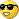Files:22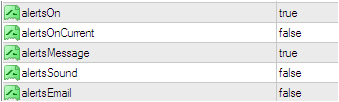Files:58

mrtools:

perfect. many thank`s.9550

Jim Clark:
Hello,

may I ask again, it is really very important for my trading !

Thanks a lot.

Jim9550

cnmfx:

can you help me again to add sound alert on this indicator?

r2_arrows_v4a2.mq4

if possible, add alerts On Current and all will be beautiful there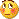I know you is the best here bross, let me know if you can help me

Regards

Cnmfx, had to make some changes to be able to add the alerts, but seems to be the same and the alerts are working.You have a choice of alerts on if Rsi above or below it's levels(the gray stars which is alertsOnRsiClose) and or for the green and red arrows(alertsOnBuyorSell).

Files: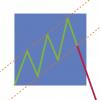108

Demos6

There still is a question : what does "to remove the main trend analyses" mean?

Sorry but I do not understand. Removing the way how it calculates would make it something different, but what should it then calculate?

A better and simpler way of explaining is: every time the oscillator reaches the 75 level and then goes down creating a peak, the oscillator draws a arrow in the main chart giving a sell signal.

And every time that the oscillator goes under de 25 level a then goes up the oscillator gives a buy signal.

If you didn't understand the previous explanation it's understandable, english is not my first language so I will try to explain using images because its easier for me.

The indicator works in this way:

1. The oscillator goes under the 25 level

2. The oscillator goes up creating a "v" shape.

Example: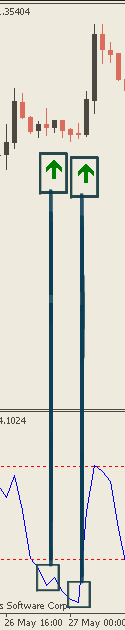To create a sell arrow.

1. The oscillator goes over the 75 level

2. The oscillator goes down creating a inverted "v" shape.

Example: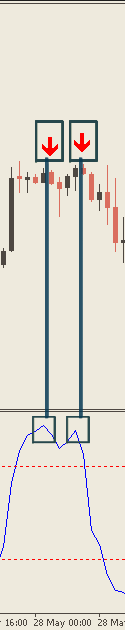But as you can see in this image that is not always the case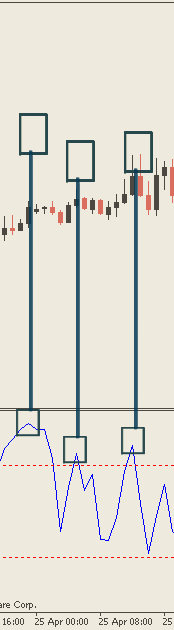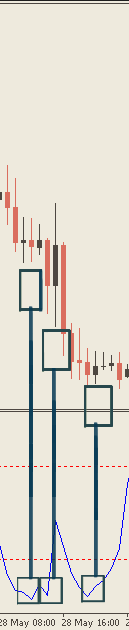this happens because this indicator uses a moving average to filter the signals in counter trend.

What I am asking is if it is possible to modify the indicator that will make the arrows appear every time independent of trend direction.

Thank you for your time and patience.9550

ratu:Ratu, have this version.

Files:587

I downloaded the Extreme RSI indicator from the forum a couple of days ago but there is no alert. i would be grateful if you would kindly add alerts for when the arrows appear - with option for alert on current candle and on close of candle. The attached indicator is what i downloaded from the forum - but when i use it i inverse the OBOS settings as follows:-

ORIGINAL SETTING

{

double r1 = iRSI(NULL,0,rsiperiod,PRICE_CLOSE,i);

double r2 = iRSI(NULL,0,rsiperiod,PRICE_CLOSE,i+1);

if (r1>30 && r2<30)

buffy1 = Low-15*Point;

bar=Time;

if (r170)

buffy2 = High+15*Point;

bar=Time;

}

REVISED SETTING

{

double r1 = iRSI(NULL,0,rsiperiod,PRICE_CLOSE,i);

double r2 = iRSI(NULL,0,rsiperiod,PRICE_CLOSE,i+1);

if (r1>70 && r2<70)

buffy1 = Low-15*Point;

bar=Time;

if (r130)

buffy2 = High+15*Point;

bar=Time;

}

I mention this just in case there is some relevance for the alerts. Thank you

extreme_rsi_1.mq4

Regards

Jasjit

Files:9550

electrical999:

I downloaded the Extreme RSI indicator from the forum a couple of days ago but there is no alert. i would be grateful if you would kindly add alerts for when the arrows appear - with option for alert on current candle and on close of candle. The attached indicator is what i downloaded from the forum - but when i use it i inverse the OBOS settings as follows:-

ORIGINAL SETTING

{

double r1 = iRSI(NULL,0,rsiperiod,PRICE_CLOSE,i);

double r2 = iRSI(NULL,0,rsiperiod,PRICE_CLOSE,i+1);

if (r1>30 && r2<30)

buffy1 = Low-15*Point;

bar=Time;

if (r170)

buffy2 = High+15*Point;

bar=Time;

}

REVISED SETTING

{

double r1 = iRSI(NULL,0,rsiperiod,PRICE_CLOSE,i);

double r2 = iRSI(NULL,0,rsiperiod,PRICE_CLOSE,i+1);

if (r1>70 && r2<70)

buffy1 = Low-15*Point;

bar=Time;

if (r130)

buffy2 = High+15*Point;

bar=Time;

}

I mention this just in case there is some relevance for the alerts. Thank you

extreme_rsi_1.mq4

Regards

Jasjit

Hi Jasjit,

Modified the indicator a bit, think possibly could have been some repainting issues, anyway this version gives the same results with the alerts.

Files: